QRコード(所蔵情報)### Numerical solutions of boundary value problems for ordinary differential equations : [proceedings of a symposium held at the University of Maryland, Baltimore County Campus, June 10-12, 1974]

フォーマット:

edited by A. K. Aziz

New York : Academic Press, 1975

ix, 369 p. : ill. ; 24 cm

BA00838535
ISBN:
9780120686605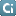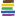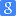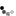タイトル・著者・出版者が同じ資料

### 類似資料:

1

2

3

4

5

6

7

8

9

10

11

12

 1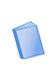図書 Conference on the Numerical Solution of Differential Equations : held in Dundee/Scotland, June 23-27, 1969 Conference on the Numerical Solution of Differential Equations, Morris, John Ll. Springer-Verlag 7図書 Numerical solution of partial differential equations : proceedings of a symposium held at the University of Maryland, College Park, Maryland, May 3-8, 1965 Symposium on the Numerical Solution of Partial Differential Equations, Blamble, James H. Academic Press 2図書 Numerical approximation of partial differential equations : selection of papers presented at the International Symposium on Numerical Analysis, held at the … International Symposium on Numerical Analysis, Ortiz, Eduardo L. North-Holland 8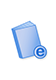電子ブック Codes for Boundary-Value Problems in Ordinary Differential Equations Childs Springer Berlin Heidelberg 3図書 Topological methods for ordinary differential equations : lectures given at the 1st session of the Centro Internazionale Matematico Estivo (C.I.M.E.), held in … Fitzpatrick, Patrick, 1946-, Furi, M. (Massimo), Zecca, P. (Pietro), Centro internazionale matematico estivo, Centro … Springer-Verlag 9図書 Numerical solution of ordinary differential equations Lapidus, Leon, Seinfeld, John H., 1942- Academic Press 4図書 Numerical methods for ordinary differential equations : proceedings of the workshop held in L'Aquila (Italy), Sept. 16-18, 1987 Bellen, A. (Alfredo), 1941-, Gear, Charles William, 1935-, Russo, E. (Elvira), 1940-, Symposium on Ordinary Differential … Springer-Verlag 10図書 Differential equations : stability and control Elaydi, Saber, 1943-, International Conference on Differential Equations: Theory and Applications in Stability and … M. Dekker 5図書 Differential equations and mathematical physics : proceedings of the international conference held at the University of Alabama at Birmingham, March 15-21, 1990 International Conference on Differential Equations and Mathematical Physics, Bennewitz, Christer, 1943- Academic Press 11図書 The numerical solution of ordinary and partial differential equations Sewell, Granville Academic Press 6図書 The numerical solution of two-point boundary problems in ordinary differential equations Fox, L. (Leslie) Clarendon Press 12図書 The numerical solution of ordinary and partial differential equations Sewell, Granville Wiley-Interscience